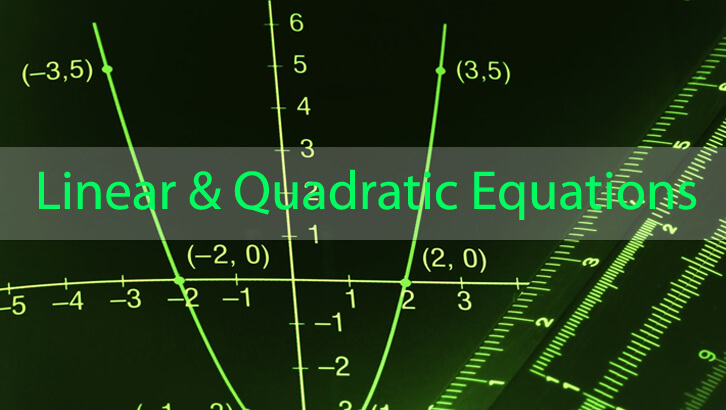# CAT Preparation Online – A simple question from Quadratic Equations

Questions

x^2 – 17x + |p| = 0 has integer solutions. How many values can p take?

Explanation

To begin with, if we assume roots to be a and b, sum of the roots is 17 and product of the roots is |p|. Product of the roots is positive and so is the sum  of the two roots.

So, both roots need to be positive.

So, we are effectively solving for

Number of positive integer solutions for a + b = 17.

We could have (1,16), (2, 15), (3, 14)……(8, 9) – There are 8 sets of pairs of roots. Each of these will yield a different product.

So, |p| can take 8 different values. Or, p can take 16 different values.

is that it? Or, are we missing something? Can p be zero? What are the roots of x^2 – 17x = 0. This equation also has integer solutions.

So, p can also be zero.

So, number of possible values of p = 16 + 1 = 17.

Wonderful question – chiefly because there are two really good wrong answers one can get. 8 and 16. So, pay attention to detail. No point telling yourself “Just missed, I just didnt think of zero. I deserve this mark”. Being just wrong, will earn us a -1 instead of the honourable 0.